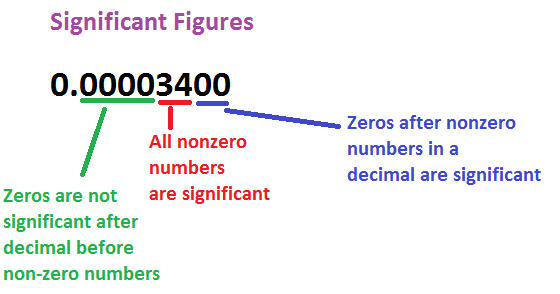# SIG FIG Calculator

## What are significant figures?

Significant figures, or sig figs for short, are the meaningful digits in a number. Often, leading zeroes or trailing zeroes can be removed and the number remains just as accurate (004 means the same as 4, for example). When removing digits, you must be able to identify the significant figures in order to retain the number’s accuracy. When you round a number up or down, one or some of the significant figures are altered.

## Sig figs calculator operators

You can use the following operators and functions with this calculator:

• Addition ( + ), subtraction ( – ), division ( / or ÷ ) and multiplication ( * or × ). Plus exponent ( ^ )
• Grouping symbols: parentheses ( )
• Functions: log, ln

Our calculator also provides a counter, showing you the number of significant figures for any calculation.

SIG FIG Calculator Add, subtract, multiply and divide significant figures. Enter numbers, scientific notation or e notation and select the operator. The calculator does the math and provides the answer rounding to the correct number of significant figures (sig figs).

You can use this calculator to verify your own calculations using significant figures.

Rounding Significant Figures has moved.

Enter whole numbers, real numbers, scientific notation or e notation. Example inputs are, 3500, 35.0056, 3.5 x 10^3 and 3.5e3.

Caution: See note regarding significant figures calculations.## What are Significant Figures?

Significant figures are the digits of a number that are meaningful in terms of accuracy or precision. These digits provide meaningful information about the precision of a calculation or measurement. CC

## Significant Figures Rules

1. Non-zero digits are always significant
2. Zeros in between non-zero digits are always significant
3. Leading zeros are never significant
4. Trailing zeros are only significant if the number contains a decimal point

## How many significant figures are there in…?

Here are some examples of significant figure calculations:

• 7 has 1 significant figure (7).
• 73 has 2 significant figures (7 and 3).
• 100 has 1 significant figure (1).
• 673 has 3 significant figures (6, 7 and 3).
• 673.52 has 5 significant figures (6, 3, 7, 5 and 2).
• 0.0637 has 3 significant figures (6, 3 and 7).
• 30.00 has 4 significant figures (3, 0, 0 and 0) and 2 decimals.
• 0.0025 has 2 significant figures (2 and 5) and 4 decimals.

### How do you calculate sig figs?

Rules for Numbers WITH a Decimal Point
1. START counting for sigfigs. On the FIRST non-zero digit.
2. STOP counting for sigfigs. …
3. Non-zero digits are ALWAYS significant.
4. Any zero AFTER the first non-zero digit is STILL significant. The zeroes BEFORE the first non-zero digit are insignificant.​​

### How many significant figures does 0.0100 have?

Trailing zeros after a decimal point count if preceded by a non-zero value. Example: 0.01 one significant figure, 0.010 two significant figures, 0.0100 three significant figures

How many significant figures does 3.00 have?

How many sig figs do I round to?

How many significant figures does 0.0560 have?

How many significant figures does 0.020 have?

How many significant digits do 10.097 have?

How many significant figures are there in 5000?

How many significant digits does 1.00 have? parcel Tracking